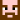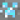Plugins alerted me, went over, confronted him, he goes “meh, just been digging”. Right:

Spoiler
[Tue Nov 06 22:26:22 HKT 2012] DIAMOND_ORE broken by bibowaddle15 at (x=  -218, y=  10, z= -1916, l= 3)
[Tue Nov 06 22:26:27 HKT 2012] DIAMOND_ORE broken by bibowaddle15 at (x=  -218, y=   9, z= -1916, l= 4)
[Tue Nov 06 22:26:42 HKT 2012] DIAMOND_ORE broken by bibowaddle15 at (x=  -217, y=  10, z= -1915, l=13)
[Tue Nov 06 22:26:42 HKT 2012] DIAMOND_ORE broken by bibowaddle15 at (x=  -217, y=   9, z= -1915, l=12)
[Tue Nov 06 22:26:42 HKT 2012] LAPIS_ORE   broken by bibowaddle15 at (x=  -218, y=   9, z= -1915, l=11)
[Tue Nov 06 22:27:22 HKT 2012] DIAMOND_ORE broken by bibowaddle15 at (x=  -233, y=  12, z= -1922, l= 4) [t=  38sec / (d= 17 / b=  10) = r=22.0] [flagged x1; ratio paranoidDiamonds]
[Tue Nov 06 22:27:22 HKT 2012] DIAMOND_ORE broken by bibowaddle15 at (x=  -233, y=  13, z= -1922, l= 3)
[Tue Nov 06 22:27:27 HKT 2012] DIAMOND_ORE broken by bibowaddle15 at (x=  -234, y=  13, z= -1922, l= 2)
[Tue Nov 06 22:27:27 HKT 2012] DIAMOND_ORE broken by bibowaddle15 at (x=  -234, y=  12, z= -1922, l= 3)
[Tue Nov 06 22:27:27 HKT 2012] DIAMOND_ORE broken by bibowaddle15 at (x=  -233, y=  13, z= -1921, l= 2)
[Tue Nov 06 22:27:27 HKT 2012] DIAMOND_ORE broken by bibowaddle15 at (x=  -233, y=  12, z= -1921, l= 3)
[Tue Nov 06 22:27:32 HKT 2012] DIAMOND_ORE broken by bibowaddle15 at (x=  -234, y=  13, z= -1921, l= 1)
[Tue Nov 06 22:27:32 HKT 2012] DIAMOND_ORE broken by bibowaddle15 at (x=  -234, y=  12, z= -1921, l= 2)
[Tue Nov 06 22:28:57 HKT 2012] EMERALD_ORE broken by bibowaddle15 at (x=  -214, y=  29, z= -1966, l= 9) [t=  88sec / (d= 52 / b=  20) = r=33.8] [flagged x2; ratio] [cave]
[Tue Nov 06 22:33:37 HKT 2012] DIAMOND_ORE broken by bibowaddle15 at (x=  -198, y=  14, z= -1948, l= 4) [t= 276sec / (d= 28 / b=  60) = r=583.7]
[Tue Nov 06 22:33:37 HKT 2012] DIAMOND_ORE broken by bibowaddle15 at (x=  -197, y=  14, z= -1948, l= 3)
[Tue Nov 06 22:33:37 HKT 2012] DIAMOND_ORE broken by bibowaddle15 at (x=  -198, y=  13, z= -1948, l= 3)
[Tue Nov 06 22:33:42 HKT 2012] DIAMOND_ORE broken by bibowaddle15 at (x=  -197, y=  13, z= -1948, l= 2)
[Tue Nov 06 22:33:42 HKT 2012] DIAMOND_ORE broken by bibowaddle15 at (x=  -197, y=  13, z= -1949, l= 1)
[Tue Nov 06 22:33:42 HKT 2012] DIAMOND_ORE broken by bibowaddle15 at (x=  -197, y=  14, z= -1949, l= 2)
[Tue Nov 06 22:34:12 HKT 2012] EMERALD_ORE broken by bibowaddle15 at (x=  -202, y=  14, z= -1946, l= 8)
[Tue Nov 06 22:38:03 HKT 2012] DIAMOND_ORE broken by bibowaddle15 at (x=  -201, y=   9, z= -1891, l= 4) [t= 232sec / (d= 55 / b=  87) = r=365.4]
[Tue Nov 06 22:38:03 HKT 2012] DIAMOND_ORE broken by bibowaddle15 at (x=  -202, y=   9, z= -1891, l= 3)
[Tue Nov 06 22:38:07 HKT 2012] DIAMOND_ORE broken by bibowaddle15 at (x=  -201, y=   8, z= -1890, l=13)
[Tue Nov 06 22:38:07 HKT 2012] DIAMOND_ORE broken by bibowaddle15 at (x=  -202, y=   8, z= -1890, l=12)
[Tue Nov 06 22:38:42 HKT 2012] DIAMOND_ORE broken by bibowaddle15 at (x=  -206, y=   4, z= -1899, l= 9) [t=  31sec / (d= 11 / b=  33) = r=96.2] [flagged x3; ratio]
[Tue Nov 06 22:38:42 HKT 2012] DIAMOND_ORE broken by bibowaddle15 at (x=  -205, y=   4, z= -1899, l= 8)
[Tue Nov 06 22:38:42 HKT 2012] DIAMOND_ORE broken by bibowaddle15 at (x=  -206, y=   3, z= -1899, l= 8)
[Tue Nov 06 22:38:47 HKT 2012] DIAMOND_ORE broken by bibowaddle15 at (x=  -205, y=   4, z= -1900, l= 9)
[Tue Nov 06 22:38:47 HKT 2012] DIAMOND_ORE broken by bibowaddle15 at (x=  -205, y=   5, z= -1900, l=10)
[Tue Nov 06 22:39:22 HKT 2012] EMERALD_ORE broken by bibowaddle15 at (x=  -199, y=   7, z= -1900, l= 6)
[Tue Nov 06 22:40:27 HKT 2012] DIAMOND_ORE broken by bibowaddle15 at (x=  -208, y=   3, z= -1916, l= 3) [t=  62sec / (d= 19 / b=  62) = r=204.6] [flagged x4; ratio]
[Tue Nov 06 22:40:27 HKT 2012] DIAMOND_ORE broken by bibowaddle15 at (x=  -207, y=   3, z= -1917, l= 1)
[Tue Nov 06 22:40:27 HKT 2012] DIAMOND_ORE broken by bibowaddle15 at (x=  -208, y=   2, z= -1917, l= 1)

This entry was posted in Bans by uncovery. Bookmark the permalink.Owner & admin of this server.

## 13 thoughts on “Banned bibowaddle15 for Xray”

1. interesting. was he in the Darklands? Looks like he hit a lucky spot to me, or he hacked it.

•Daiyamondo on said:

It says “X-ray”.

2.VixenGold on said:

I have been underground several times in the new Darklands looking for diamonds. Out of a weeks worth of work I found 2 pockets of diamonds. There are a lot less diamonds in the new then the old. He found all those diamonds in 15 minutes. To find that many diamonds that fast is defiantly a hack. There is also less gold. More iron, coal and redstone. Does not surprise me if more people use x-ray now. But it is still wrong. There is no honor in using cheats. If you are going to use x-ray then use it on a single player. Better yet if you are to lazy to honestly mine for your ore then go get a flatland lot. Then you can have all the diamonds you want.
People are real stupid if they think they can get away with using x-ray. Uncovery has stuff working that tracks what people dig up and the exact location. This is not the first time Uncovery has posted a spoiler to show what was dug. One must go back a bit to see the posts but it is there. Never think that you can cheat the game and get away with it. Uncovery will see every time.
I have looked on YouTube to see clips of people using x-ray. It is beautiful to look at on the video but would not use. Once one starts using hacks they might at well admit that they lost the game and go play something else. Not only do you cheat everyone that plays but you are cheating yourself also.
Yes mother mode is on. :)

• Bam! Harangued again!

3. Tsk..Tsk..Tsk… :I

4. That ruins the game.

5. X-ray just goes against the purpose of the game.. Diamonds are meant to be a nice surprise :)

6. Just a warning: I have a bunch of diamond ore from a contest i got and ill be using a fortune pick to break them. i wanted to say i am not using xray if it looks strange that i just broke 20 or so diamond ores XD

• Not contest, lottery. My mistake

7. The ban a day is looking pretty good.

• A promotion a day, a ban a day! :P

8. always thought it was funny that it says ratio paranoid diamonds.
Its ok diamonds! Calm down.